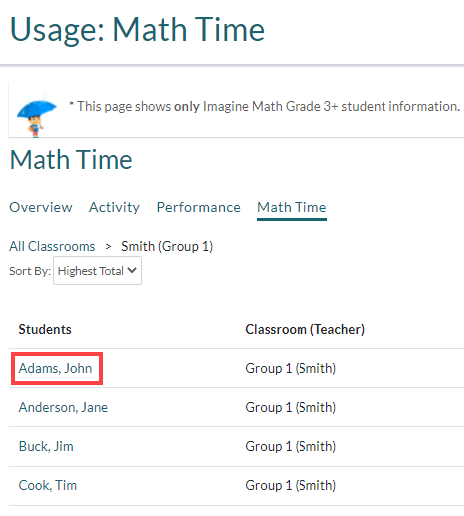# Viewing the Math Time Report in Imagine Math Grade 3+

The Math Time report shows how much time students have spent using Imagine Math Grade 3+. Administrators and Educators can view Math Time reports for any classroom or student that is assigned to them and has completed math work. Students must have answered at least 1 math problem in Imagine Math Grade 3+ in order to be counted as students with math work.

Administrators or Educators who are assigned to more than 1 classroom see the Classroom Math Time Report, which shows Math Time for each classroom. They can drill down into each classroom to see the Student Math Time Report, which shows Math Time for each student in that classroom. Administrators and Educators who are assigned to 1 classroom see the Student Math Time Report for their classroom.

Math Time accumulates when a student is actively working on a math problem or Benchmark Test question. Math Time is tracked using an internal timer and begins once a student is shown a math problem or test question. Once the student has completed the problem or question and clicked Next, Imagine Math Grade 3+ saves the time spent on that problem or question and resets the internal timer. The internal timer tracks Math Time until the student exits Imagine Math Grade 3+ or until they have been idle for 10 minutes. The Math Time Report updates hourly.

Note

Any time that students spend on the homepage, redeeming points, or loading a math problem or test question does not count towards their Total Math Time.

Note

If a student is working on a math problem or test question and does not complete it before logging out of Imagine Math Grade 3+, the time they spent on this problem or question will not be added to their Total Math Time.

To view the Math Time Report in Imagine Grade Math 3+

1. Log in to the Math Suite. If this is your first time logging in, click Imagine Math.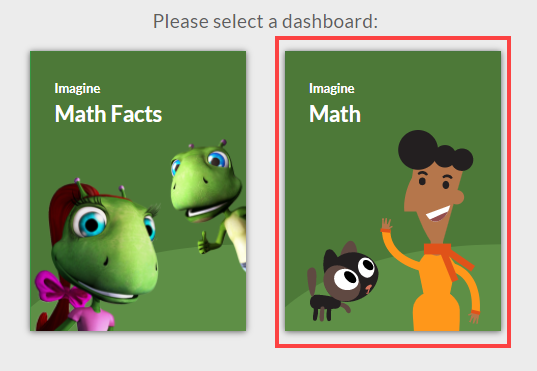2. Click Reports > Usage from the navigation pane.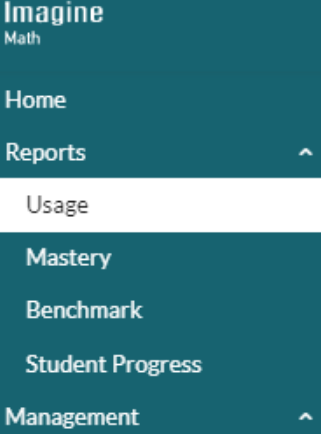3. Click the Math Time tab on the Usage Report.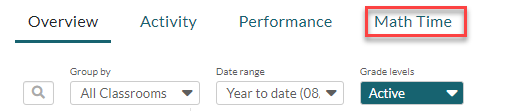If you are assigned to more than one classroom, you'll see the Classroom Math Time Report. The Classroom Math Time Report shows the classrooms that are assigned to you, the number of students in each classroom who have completed math work, the average amount of School Time and Evening/Weekend Time that the classroom has accumulated, and the total average Math Time for each classroom.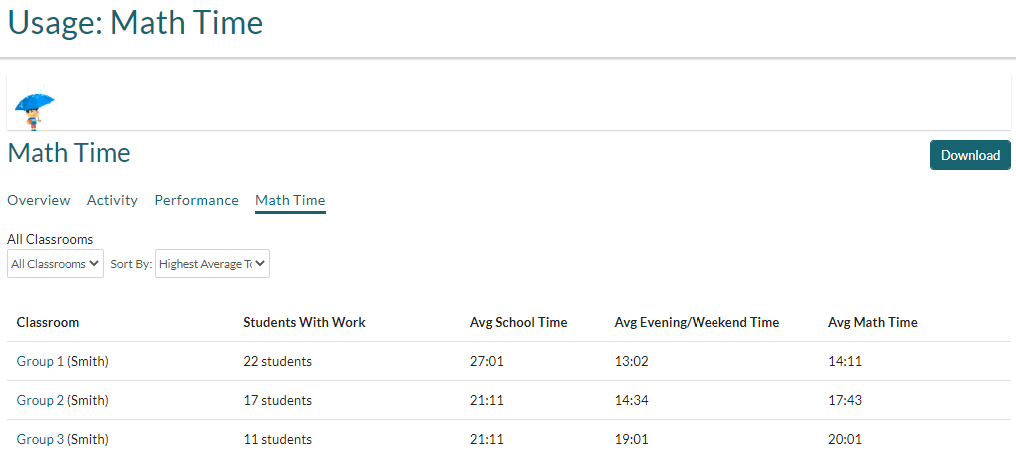4. Click the classroom that you want to see individual student Math Time for.  You can filter the Math Time Report by Classroom and sort data by Highest Average Time or Lowest Average Time. Click Download to download a CSV file of the report to print or save.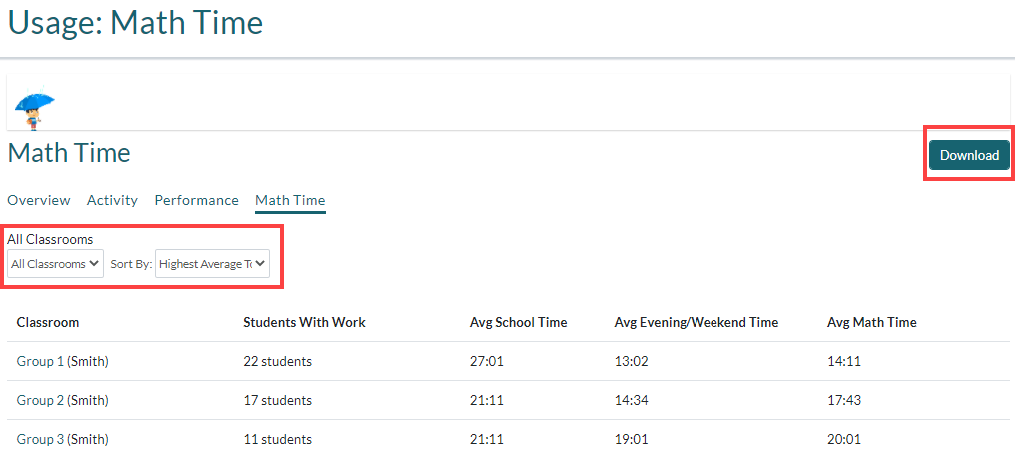The Student Math Time Report shows which classroom (teacher) each student is assigned to, the amount of School Time and Evening/Weekend Time that each student has accumulated, and the Total Math Time for each student.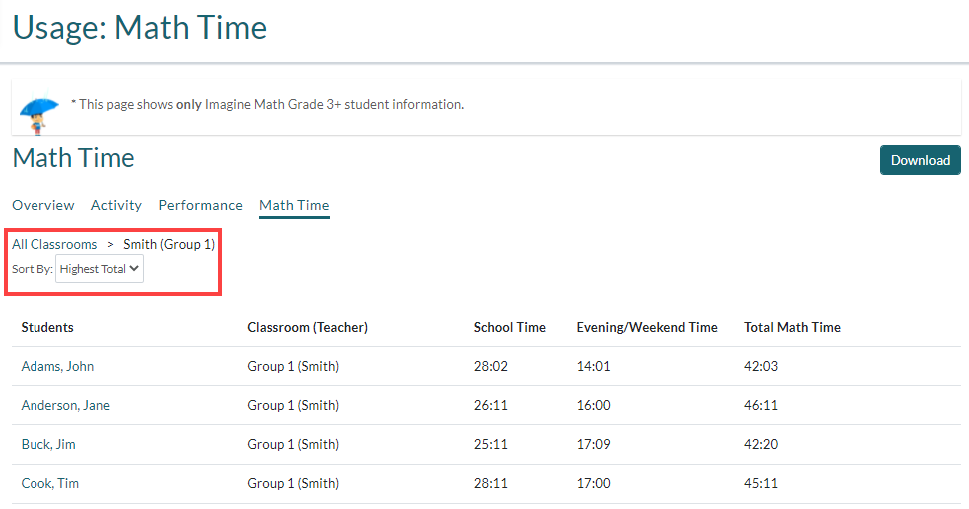Field Description
Students The student's first and last name in Imagine Math. Click on the student's name to view a Progress Report for that student.
Classroom (Teacher) The classroom (teacher) that the student is assigned to.
School Time The amount of time (HH:MM) this student used Imagine Math Grade 3+ during school hours (between 7 am–4 pm local time, Monday– Friday).
Evening/Weekend Time The amount of time (HH:MM) this student used Imagine Math Grade 3+ outside of school hours (between 4 pm–7 am local time, Monday–Friday, and all day Saturday–Sunday).
Total Math Time The total amount of time (HH:MM) this student used Imagine Math Grade 3+, including School and Evening/Weekend Time.
5. If you want to, click the student name to view a Progress Report for that student.